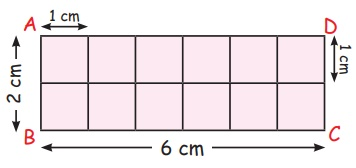Home | | Maths 5th Std | Area of the rectangle and square

# Area of the rectangle and square

Formula for the area of a rectangle. , Formula for the area of a square., Example and solution for area of a rectangle, area of a square.

Area of the rectangle and square

Situation

There are lot of mosquitoes in Kavitha's house. These mosquitoes enter her house through the windows. Kavitha's mother decided to cover the windows by a mesh. What kind of measurement is required by Kavitha's mother to cover the window using the mesh?

The measurement required by Kavitha's mother to cover the window is the area.

Area can be defined as the space occupied by a flat shape or the surface of an object.

The unit denoted for the area of an object is square units. (Example: 16 sq. cm, 24 sq. m).

Formula for the area of a rectangleFormulas

Area of the rectangle = l × b

Area of the square = a2

In the rectangle ABCD given above, 1 cm divisions were marked on each side.The points on opposite sides were joined as shown in the figure above. The length of the sides of each square thus created is 1 cm.

Therefore, the area of each square is 1 sq.cm, which is the unit square.

In the rectangle ABCD we have created 2 rows of 6 squares each.

Therefore, the number of squares in the rectangle ABCD is 6 × 2 = 12

Here, the length of the rectangle ABCD is 6 cm and its breadth is 2 cm.

The number of unit squares formed are the same as the product of the length and breadth of the rectangle.

Therefore, the area of rectangle ABCD = 6 × 2 = 12 sq.cm

Therefore, the area of the rectangle = length × breadth

Formula for the area of a square:In the square ABCD given above, 1 cm divisions were marked on each side.The points on opposite sides were joined as shown in the figure above. In the square ABCD we have created 4 rows of 4 squares each. The length of the sides of each square thus created is 1 cm.

Therefore, the area of each square is 1 sq.cm, which is the unit square.

Look at the square given above. The side of the square is 4 cm and we have 16 squares of 1cm each within this square.

The number of unit squares formed are the same as the product of two sides of the square.

Since the length of each side of the square is 4 cm the area of the square = 4 × 4 = 16 sq.cm.

Therefore, the area of a square = length of a side × length of a side

It is not necessary to divide a square or rectangle into small squares every time when you calculate their area. The advantage of a formula is that you can calculate the area simply by substituting the appropriate values.

Example 1.5

What is the area of a rectangle of length 10 cm and breadth 8 cm?

Solution

Area of a rectangle = length × breadth

= 10 × 8

= 80

Therefore, the area of the rectangle is 80 sq.cm.

Example 1.6

A wall that is 6 m long and 2 m wide has to be painted. If the labour charges are ₹ 20 per sq.m, then what is the cost of labour for painting the wall?

Solution

First let us calculate the area of the wall to be painted

Area of the wall = length of the wall × breadth of the wall

= 6 × 2 = 12

Thus, the area of the wall is 12 sq.m.

Labour cost of 1 sq.m is ₹ 20.

So, the labour cost for 12 sq.m. = 12 × 20 = ₹ 240.

Therefore, the cost of labour for painting the wall is ₹ 240.

Example 1.7

What is the area of a square with side 12 cm?

Solution

Area of a square = length of a side × length of a side

= 12 × 12 = 144

Therefore, the area of the square is 144 sq.cm.

Example 1.8

One side of a square room is 3 m. If the cost of labour for laying 1 sq.m of the floor is ₹ 25, then what is the total cost of labour?

Solution

First we must find the area of the square room

Area of the square room = length of a side × length of a side

= 3 × 3 = 9

Therefore, the area of the square room is 9 sq. m.

The labour cost of laying 1 sq.m of flooring is ₹25.

Therefore, the cost of laying 9 sq.m of flooring is 25 × 9 = ₹ 225.

Tags : Geometry | Term 3 Chapter 1 | 5th Maths , 5th Maths : Term 3 Unit 1 : Geometry
Study Material, Lecturing Notes, Assignment, Reference, Wiki description explanation, brief detail
5th Maths : Term 3 Unit 1 : Geometry : Area of the rectangle and square | Geometry | Term 3 Chapter 1 | 5th Maths